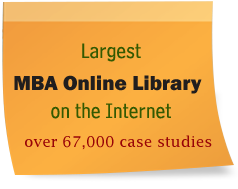### 3 Risk And Capital

1. The risk-free rate of return (Krf) is the rate of return that investors demand from a project that contains no risk (Gallagher & Andrew). In order to find this amount, we must go to Bloomberg.com and find the current rate for today, July 28, 2006, which happens to be 5.05% (Bloomberg.com). This means that 5.05% is the least that an investor will accept for a return on their investment.
2. The values for IBM stocks that are given in the stock information document that accompanied this assignment:
IBM's beta (â average = 1) = 1.64
IBM's current annual dividend = \$0.80 per share
IBM's 3-year dividend growth rate (g) = 8.20%
Industry P/E = \$23.20
IBM's EPS (net income/shares) = \$4.87
3. The CAPM formula and the interpretation of its components are as follows:
kp = kRF + (kM ? kRF) x â
In this formula, the capital asset pricing model, we calculate the required rate of return on an investment project given its degree of risk as measured by beta. So the meanings of each component of this formula are as follows:
kp = the measured rate of return suitable for the investment project
kRF = the risk-free rate of return
kM = the required rate of return on the overall market
â = the project's beta
So by inserting our quantities that we have already found we can find the answer to our CAPM formula for this problem. We must as remember to use the 5.00 as our risk-free rate of return, which was the answer we found for question 1. We must also use the assumed market risk premium of 7.5%, which was provided in the text of this assignment. Since our Km-Krf is equal to the market risk premium, we can therefore find our value using the premium rate we were already given. Below is our calculation once the numbers are plugge ...
1135 Word (s) : Pages (s) : View (s) : Rank : Report this paper